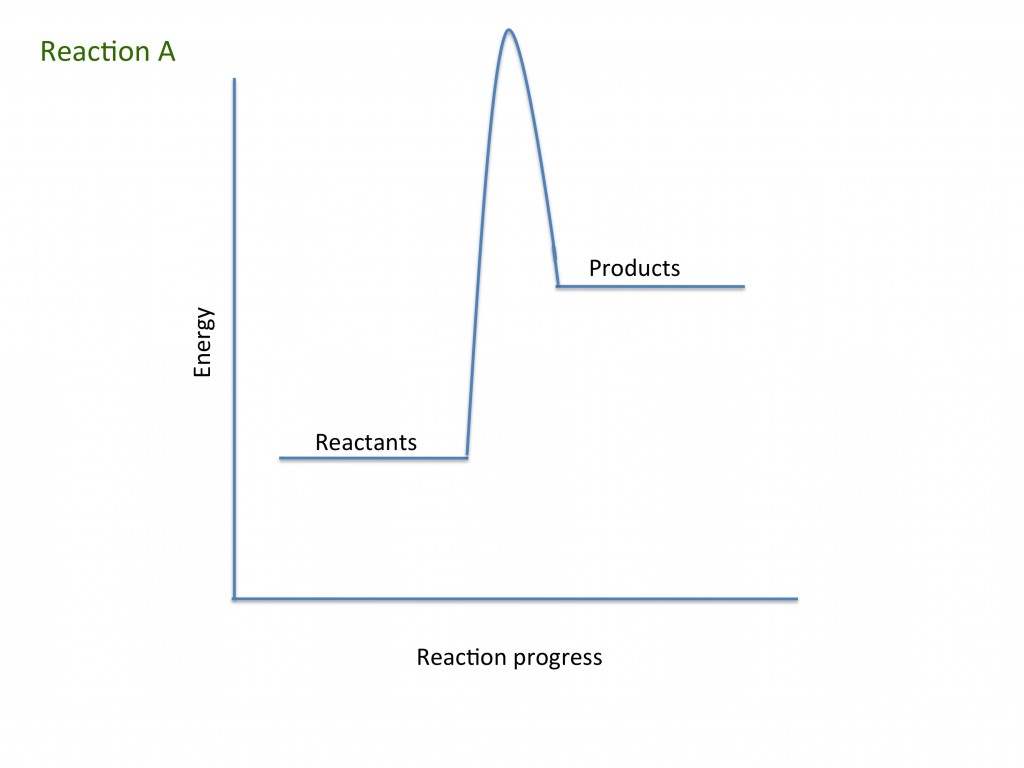# Rates of reaction teaching resources

###### Worksheets and lesson ideas to challenge students to think hard about rates of reaction, graphs and collision theory (GCSE and Key Stage 3)Rates of reaction is a rich topic area for practicals that students really enjoy. When teaching this for the first time, it is important to stress that a rate is the change in the amount of a reactant/product in a specific amount of time.

Drawing graphs (and tangents) of results from simple rate experiments can help students understand that rates involve two changing components.

The collision theory is introduced to explain experimental findings, but this is an area students can find challenging. The use of demonstrations can help students understand the important role of catalysts and activation energy in chemical reactions.

#### Thinking deeply about rates of reaction

GCSE thinking questions on rates of reaction. Students think deeply about rates of reaction and re-evaluate what they already know about rates.

Top